# Evolutionary Computing Chapter 12 Chapter 12 Multiobjective Evolutionary

• Slides: 27
Download presentationEvolutionary Computing Chapter 12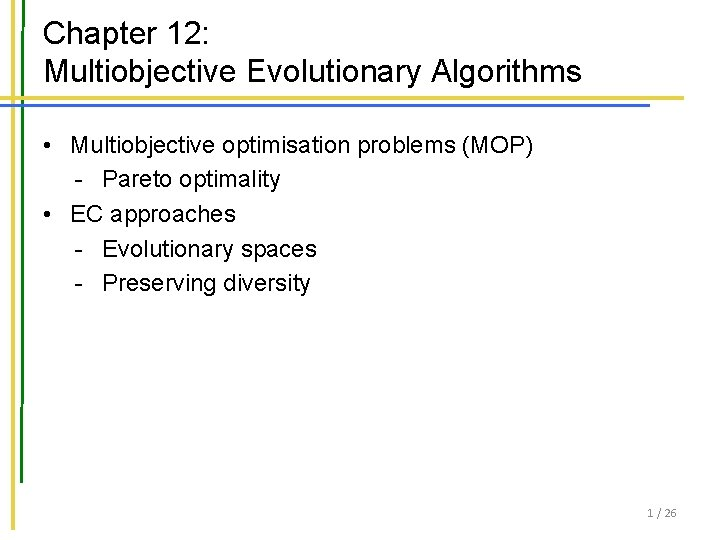Chapter 12: Multiobjective Evolutionary Algorithms • Multiobjective optimisation problems (MOP) - Pareto optimality • EC approaches - Evolutionary spaces - Preserving diversity 1 / 26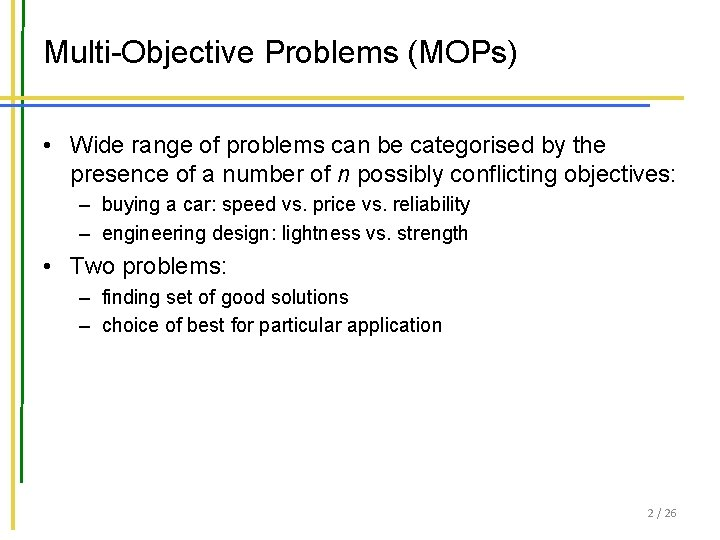Multi-Objective Problems (MOPs) • Wide range of problems can be categorised by the presence of a number of n possibly conflicting objectives: – buying a car: speed vs. price vs. reliability – engineering design: lightness vs. strength • Two problems: – finding set of good solutions – choice of best for particular application 2 / 26An example: Buying a car speed cost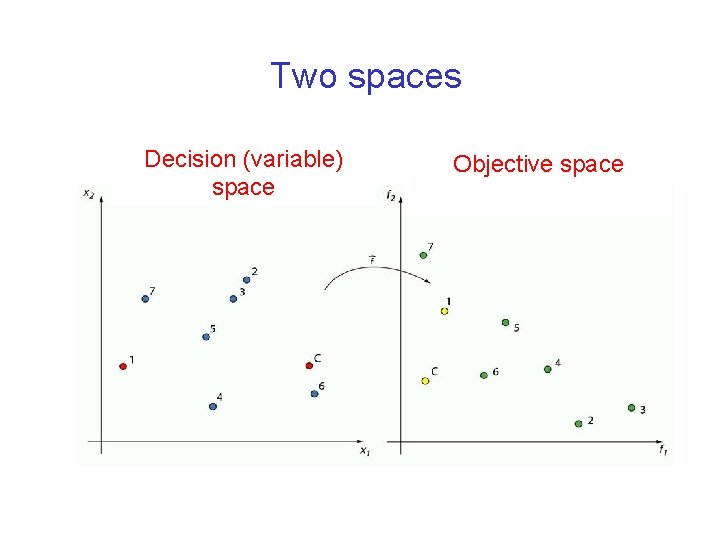Two spaces Decision (variable) space Objective space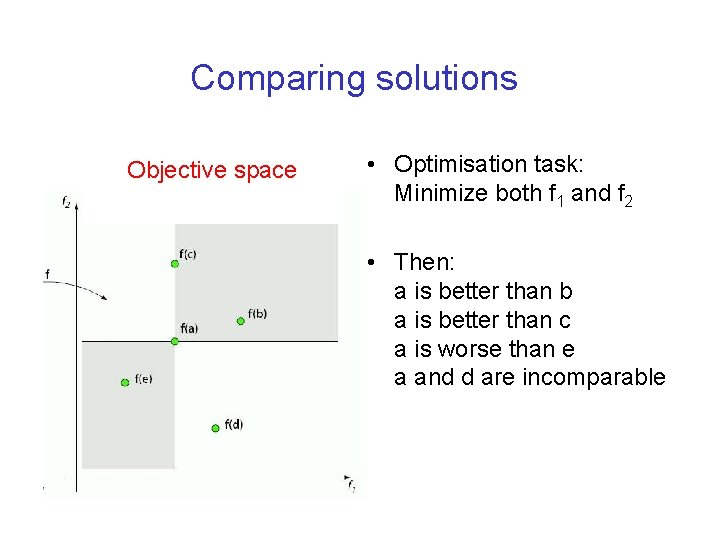Comparing solutions Objective space • Optimisation task: Minimize both f 1 and f 2 • Then: a is better than b a is better than c a is worse than e a and d are incomparableDominance relation • Solution x dominates solution y, (x y), if: – x is better than y in at least one objective, – x is not worse than y in all other objectives solutions dominated by x solutions dominating x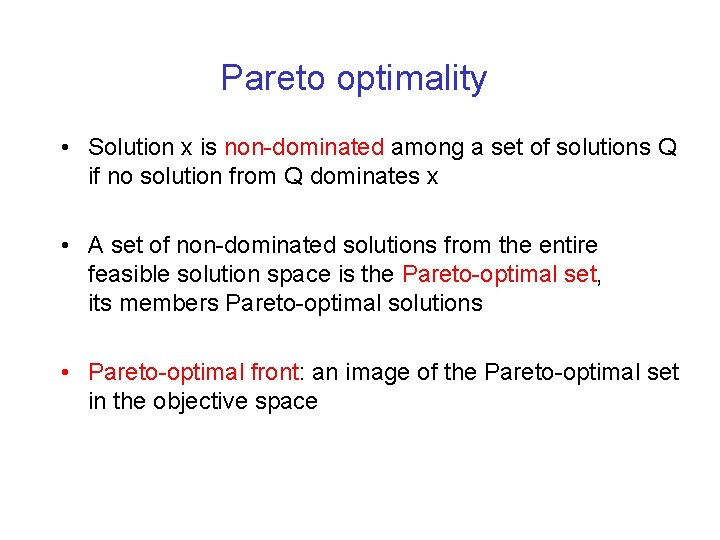Pareto optimality • Solution x is non-dominated among a set of solutions Q if no solution from Q dominates x • A set of non-dominated solutions from the entire feasible solution space is the Pareto-optimal set, its members Pareto-optimal solutions • Pareto-optimal front: an image of the Pareto-optimal set in the objective space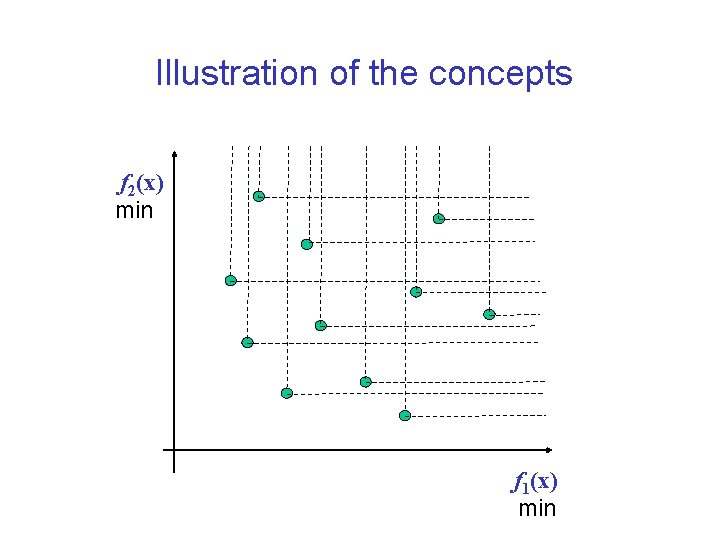Illustration of the concepts f 2(x) min f 1(x) min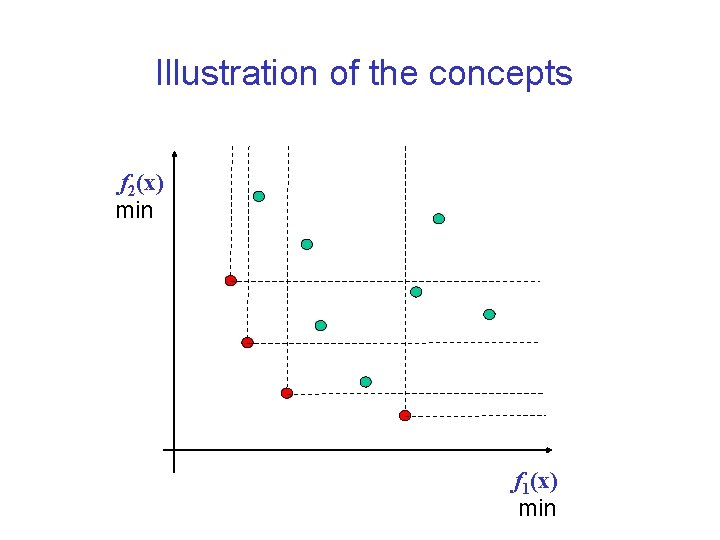Illustration of the concepts f 2(x) min f 1(x) minA practical example: The beam design problem Minimize weight and deflection of a beam (Deb, 2001): d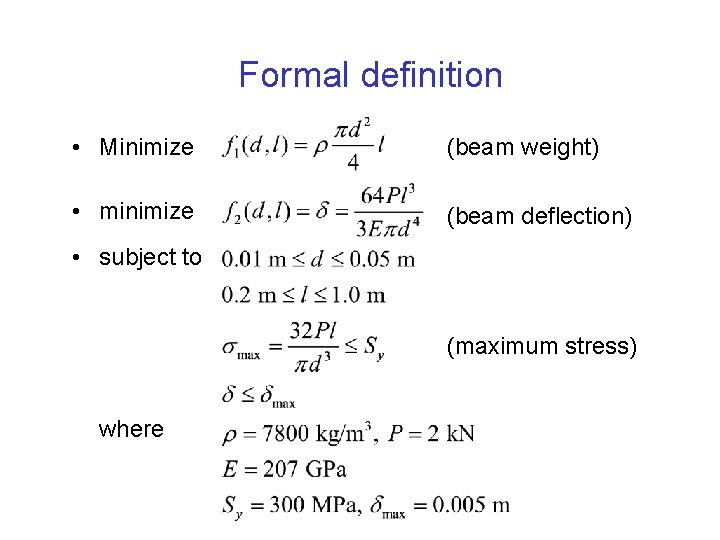Formal definition • Minimize (beam weight) • minimize (beam deflection) • subject to (maximum stress) where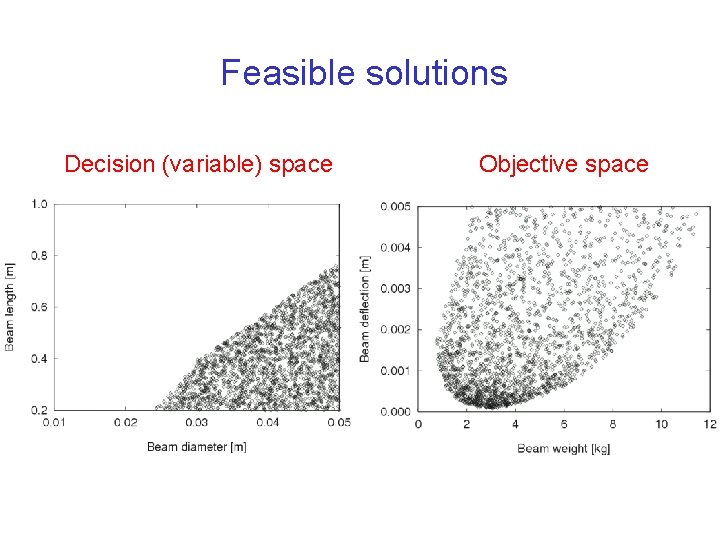Feasible solutions Decision (variable) space Objective space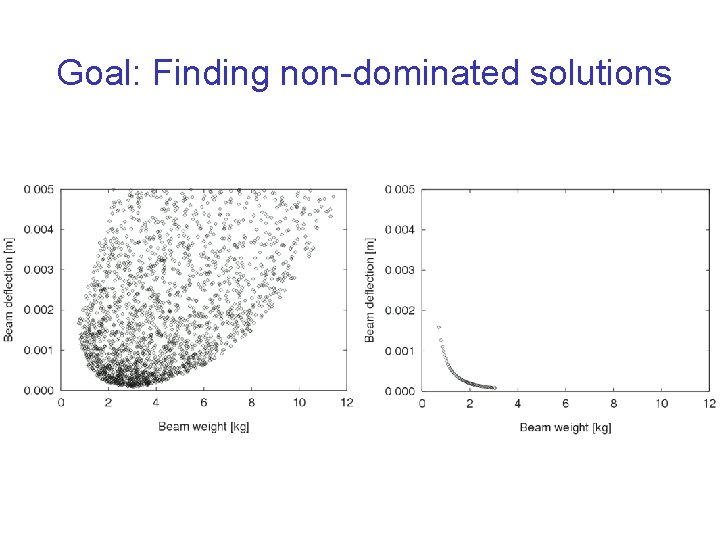Goal: Finding non-dominated solutions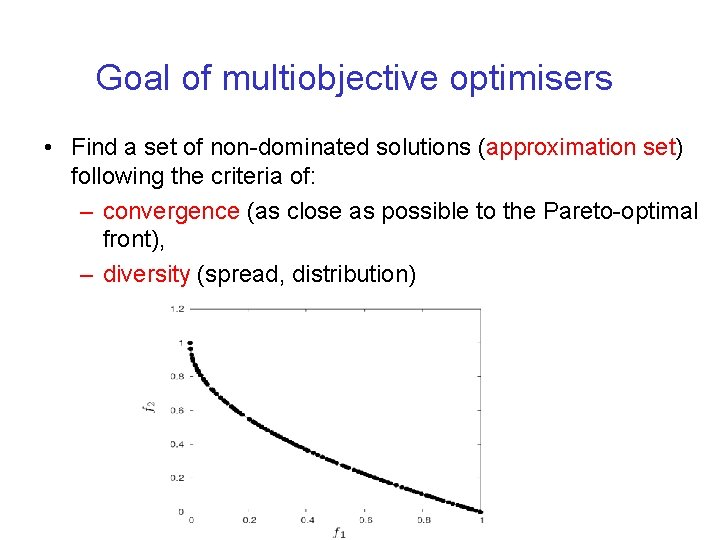Goal of multiobjective optimisers • Find a set of non-dominated solutions (approximation set) following the criteria of: – convergence (as close as possible to the Pareto-optimal front), – diversity (spread, distribution)Single- vs. multiobjective optimisation Characteristic Singleobjective optimisation Multiobjective optimisation Number of objectives one more than one Spaces single two: decision (variable) space, objective space Comparison of candidate solutions x is better than y x dominates y Result one (or several equally Pareto-optimal set good) solution(s) Algorithm goals convergence, diversity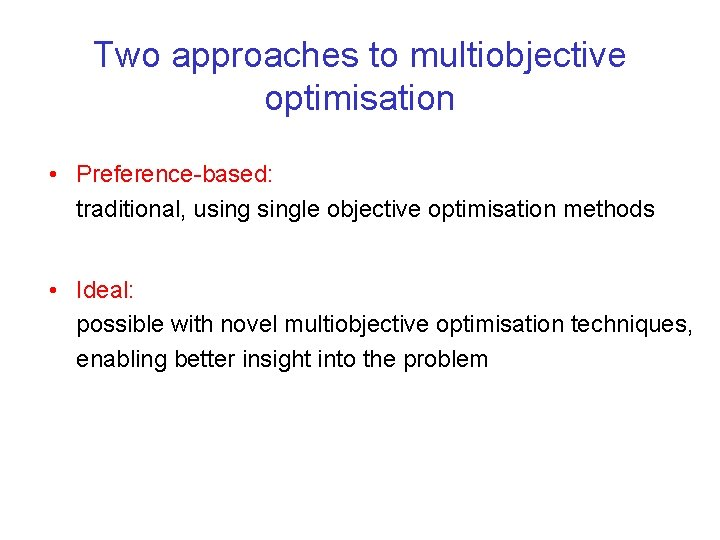Two approaches to multiobjective optimisation • Preference-based: traditional, usingle objective optimisation methods • Ideal: possible with novel multiobjective optimisation techniques, enabling better insight into the problem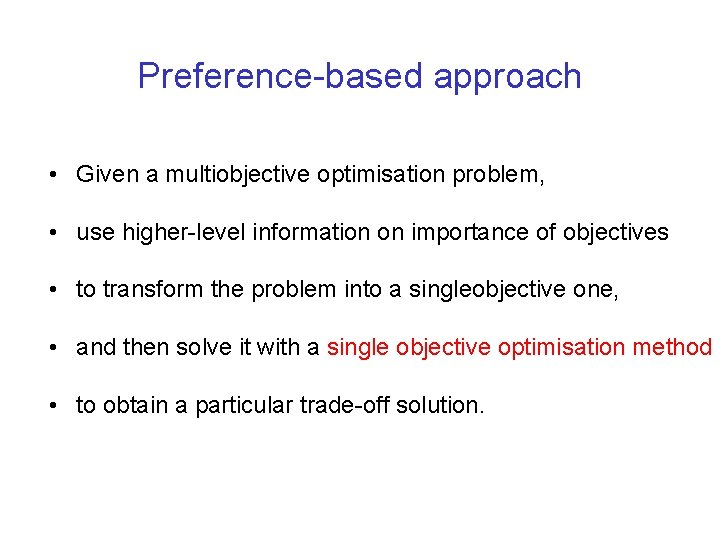Preference-based approach • Given a multiobjective optimisation problem, • use higher-level information on importance of objectives • to transform the problem into a singleobjective one, • and then solve it with a single objective optimisation method • to obtain a particular trade-off solution.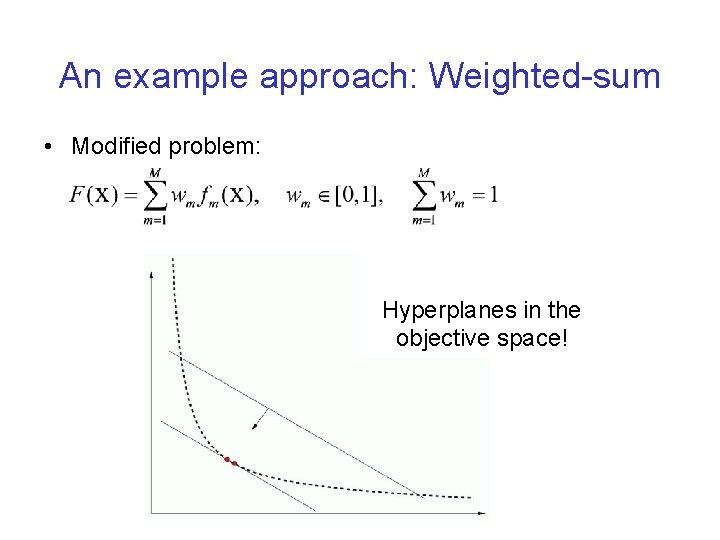An example approach: Weighted-sum • Modified problem: Hyperplanes in the objective space!Ideal approach • Given a multiobjective optimisation problem, • solve it with a multiobjective optimisation method • to find multiple trade-off solutions, • and then use higher-level information • to obtain a particular trade-off solution.Multiobjective optimisation with evolutionary algorithms • Population-based method • Can return a set of trade-off solutions (approximation set) in a single run • Allows for the ideal approach to multiobjective optimisationEC approach: Advantages • Population-based nature of search means you can simultaneously search for set of points approximating Pareto front • Don’t have to make guesses about which combinations of weights might be useful • Makes no assumptions about shape of Pareto front - can be convex / discontinuous etc. 21 / 26EC approach: Requirements • Way of assigning fitness, – usually based on dominance • Preservation of diverse set of points – similarities to multi-modal problems • Remembering all the non-dominated points you have seen – usually using elitism or an archive 22 / 26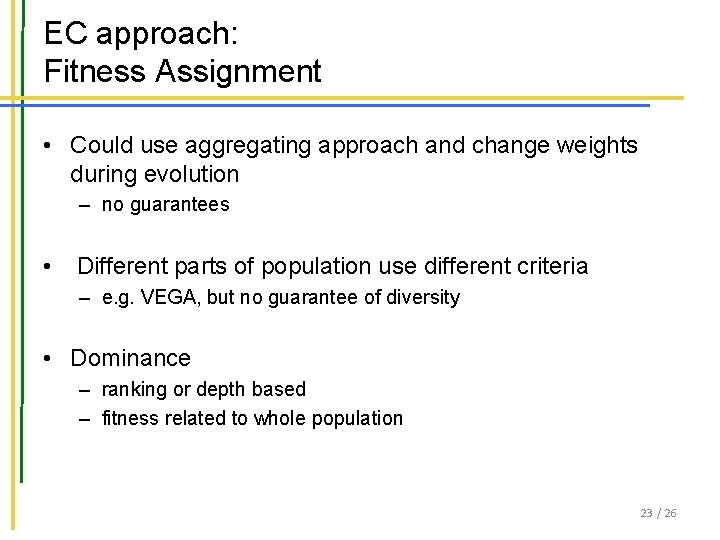EC approach: Fitness Assignment • Could use aggregating approach and change weights during evolution – no guarantees • Different parts of population use different criteria – e. g. VEGA, but no guarantee of diversity • Dominance – ranking or depth based – fitness related to whole population 23 / 26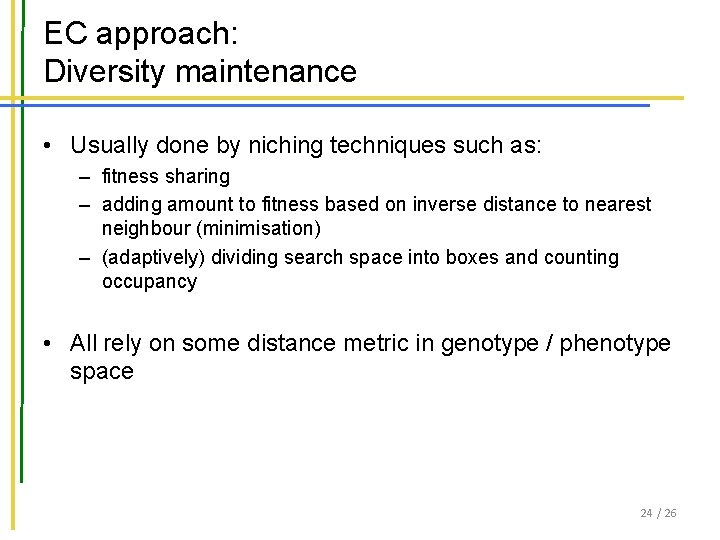EC approach: Diversity maintenance • Usually done by niching techniques such as: – fitness sharing – adding amount to fitness based on inverse distance to nearest neighbour (minimisation) – (adaptively) dividing search space into boxes and counting occupancy • All rely on some distance metric in genotype / phenotype space 24 / 26EC approach: Remembering Good Points • Could just use elitist algorithm – e. g. ( + ) replacement • Common to maintain an archive of non-dominated points – some algorithms use this as second population that can be in recombination etc. – others divide archive into regions too, e. g. PAES 25 / 26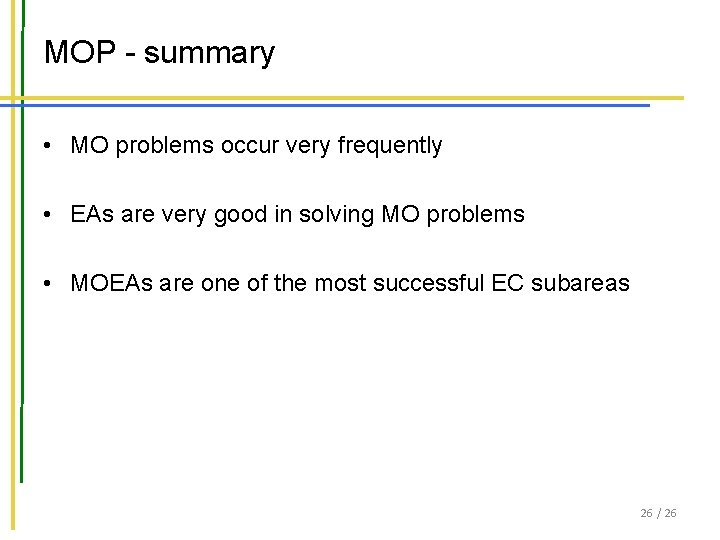MOP - summary • MO problems occur very frequently • EAs are very good in solving MO problems • MOEAs are one of the most successful EC subareas 26 / 26